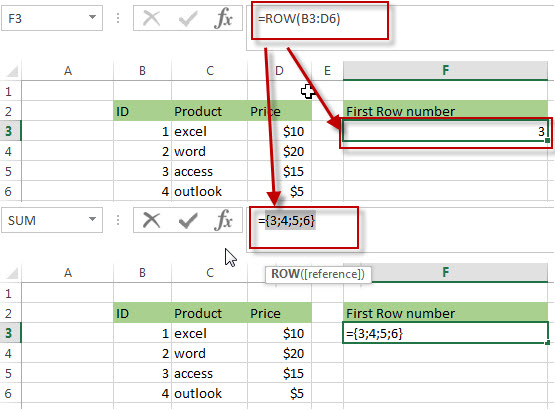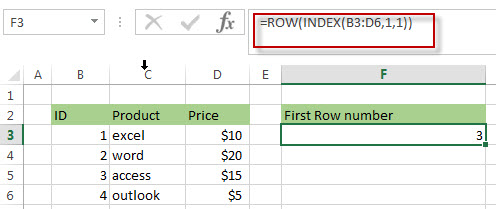# Three Ways to get the First Row Number in a Range

This post explains that how to get the first row number in a range in excel. This post will guide how to get the first row number of a range via the below three ways:

## Get First Row Number using the ROW Function Only

If the ROW function use a Range as its argument, it only returns the first row number. Actually, it returns an array contain all row numbers as elements, but it just only display one value that it is the first row number. So you can use it to get the first row number in a range directly.

Assuming that you want to get the first row number in range B3:D6, just enter the below formula into the cell F3:

`=ROW(B3:D6)`In Cell F3, the above formula returns 3, it indicated that the first row number is 3 in the range B3:D6.

## Get First Row Number using the Row Function within MIN Function

You can also use the ROW function within the MIN function to get the first row number in a range.  Like I said above, the ROW function actually return an array, so you can use MIN function to get the minimum value in the array. For example, to get the first row number of range B3:D6 using the following formula:

`=MIN(ROW(B3:D6))`

## Get First Row Number using the Row function within INDEX Function

You can also use the INDEX function to get the reference of the first row in a range, then combined to the ROW function to get the first row number of a range. Just try the following formula:

=ROW(INDEX(B3:D6,1,1))

The INDEX function returns the reference at a given position based on Row_num and Col_num arguments. So the INDEX(B3:D6,1,1) formula will return the reference of the first row in the range B3:D6.### Related Formulas

• Combine Text from Two or More Cells into One Cell
If you want to combine text from multiple cells into one cell and you can use the Ampersand (&) symbol.If you are using the excel 2016, then you can use a new function TEXTJOIN function to combine text from multiple cells…
• Split Text String to an Array
If you want to convert a text string into an array that split each character in text as an element, you can use an excel formula to achieve this result. the below will guide you how to use a combination of the MID function, the ROW function, the INDIRECT function and the LEN function to split a string…
• Find the Relative Position in a Range or Table
If you want to know the relative row position for all rows in an Excel Range (B3:D6), you can use a excel Array formula as follows:=ROW(B3:D6)- ROW(B3) + 1. You can also use another excel array formula to get the same result as follows:=ROW(B3:D6)-ROW(INDEX(B3:D6,1,1))+1…
•  Get the Last Row Number in a Range
If you want to get the last row number in a range, you need to know the first row number and the total rows number of a range, then perform the addition operation, then subtract 1, the last result is the last row number for that range.…

### Related Functions

• Excel INDEX function
The Excel INDEX function returns a value from a table based on the index (row number and column number)The INDEX function is a build-in function in Microsoft Excel and it is categorized as a Lookup and Reference Function.The syntax of the INDEX function is as below:= INDEX (array, row_num,[column_num])…
• Excel ROW function
The Excel ROW function returns the row number of a cell reference.The ROW function is a build-in function in Microsoft Excel and it is categorized as a Lookup and Reference Function.The syntax of the ROW function is as below:= ROW ([reference])….
• Excel MIN function
The Excel MIN function returns the smallest numeric value from the numbers that you provided. Or returns the smallest value in the array.The MIN function is a build-in function in Microsoft Excel and it is categorized as a Statistical Function.The syntax of the MIN function is as below:= MIN(num1,[num2,…numn])….